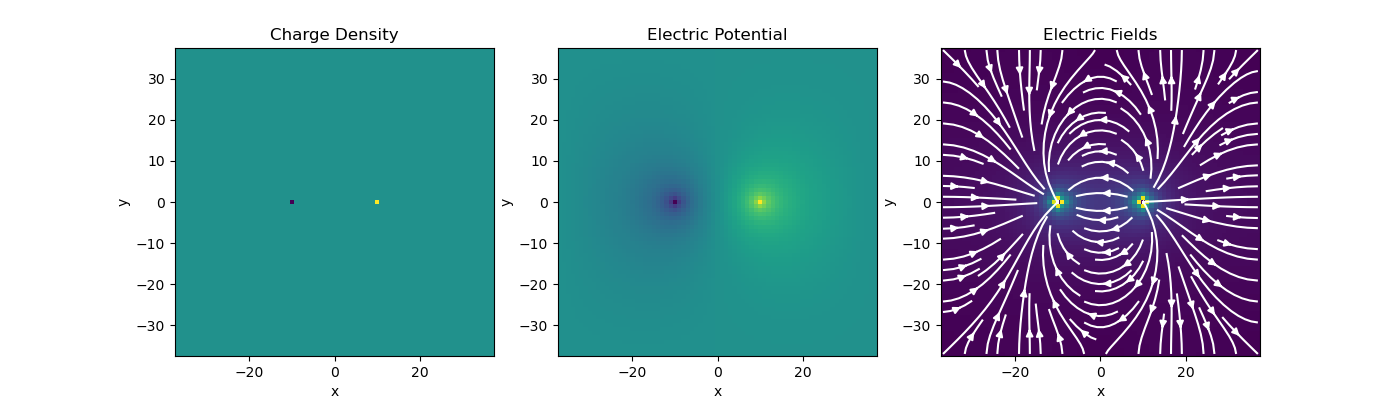# Gauss’ Law of Electrostatics#

Here we use the discretize package to solve for the electric potential ($$\phi$$) and electric fields ($$\mathbf{e}$$) in 2D that result from a static charge distribution. Starting with Gauss’ law and Faraday’s law:

\begin{align}\begin{aligned}&\nabla \cdot \mathbf{E} = \frac{\rho}{\epsilon_0}\\&\nabla \times \mathbf{E} = \mathbf{0} \;\;\; \Rightarrow \;\;\; \mathbf{E} = -\nabla \phi\\&\textrm{s.t.} \;\;\; \phi \Big |_{\partial \Omega} = 0\end{aligned}\end{align}

where $$\sigma$$ is the charge density and $$\epsilon_0$$ is the permittivity of free space. We will consider the case where there is both a positive and a negative charge of equal magnitude within our domain. Thus:

$\rho = \rho_0 \big [ \delta ( \mathbf{r_+}) - \delta (\mathbf{r_-} ) \big ]$

To solve this problem numerically, we use the weak formulation; that is, we take the inner product of each equation with an appropriate test function. Where $$\psi$$ is a scalar test function and $$\mathbf{f}$$ is a vector test function:

\begin{align}\begin{aligned}\int_\Omega \psi (\nabla \cdot \mathbf{E}) dV = \frac{1}{\epsilon_0} \int_\Omega \psi \rho dV\\\int_\Omega \mathbf{f \cdot E} \, dV = - \int_\Omega \mathbf{f} \cdot (\nabla \phi ) dV\end{aligned}\end{align}

In the case of Gauss’ law, we have a volume integral containing the Dirac delta function, thus:

$\int_\Omega \psi (\nabla \cdot \mathbf{E}) dV = \frac{1}{\epsilon_0} \psi \, q$

where $$q$$ represents an integrated charge density. By applying the finite volume approach to this expression we obtain:

$\mathbf{\psi^T M_c D e} = \frac{1}{\epsilon_0} \mathbf{\psi^T q}$

where $$\mathbf{q}$$ denotes the total enclosed charge for each cell. Thus $$\mathbf{q_i}=\rho_0$$ for the cell containing the positive charge and $$\mathbf{q_i}=-\rho_0$$ for the cell containing the negative charge. It is zero for every other cell.

$$\mathbf{\psi}$$ and $$\mathbf{q}$$ live at cell centers and $$\mathbf{e}$$ lives on cell faces. $$\mathbf{D}$$ is the discrete divergence operator. $$\mathbf{M_c}$$ is an inner product matrix for cell centered quantities.

For the second weak form equation, we make use of the divergence theorem as follows:

\begin{align}\begin{aligned}\int_\Omega \mathbf{f \cdot E} \, dV &= - \int_\Omega \mathbf{f} \cdot (\nabla \phi ) dV\\& = - \frac{1}{\epsilon_0} \int_\Omega \nabla \cdot (\mathbf{f} \phi ) dV + \frac{1}{\epsilon_0} \int_\Omega ( \nabla \cdot \mathbf{f} ) \phi \, dV\\& = - \frac{1}{\epsilon_0} \int_{\partial \Omega} \mathbf{n} \cdot (\mathbf{f} \phi ) da + \frac{1}{\epsilon_0} \int_\Omega ( \nabla \cdot \mathbf{f} ) \phi \, dV\\& = 0 + \frac{1}{\epsilon_0} \int_\Omega ( \nabla \cdot \mathbf{f} ) \phi \, dV\end{aligned}\end{align}

where the surface integral is zero due to the boundary conditions we imposed. Evaluating this expression according to the finite volume approach we obtain:

$\mathbf{f^T M_f e} = \mathbf{f^T D^T M_c \phi}$

where $$\mathbf{f}$$ lives on cell faces and $$\mathbf{M_f}$$ is the inner product matrix for quantities that live on cell faces. By canceling terms and combining the set of discrete equations we obtain:

$\big [ \mathbf{M_c D M_f^{-1} D^T M_c} \big ] \mathbf{\phi} = \frac{1}{\epsilon_0} \mathbf{q}$

from which we can solve for $$\mathbf{\phi}$$. The electric field can be obtained by computing:

$\mathbf{e} = \mathbf{M_f^{-1} D^T M_c \phi}$

## Import Packages#

Here we import the packages required for this tutorial.

from discretize import TensorMesh
from pymatsolver import SolverLU
import matplotlib.pyplot as plt
import numpy as np
from discretize.utils import sdiag


## Solving the Problem#

# Create a tensor mesh
h = np.ones(75)
mesh = TensorMesh([h, h], "CC")

# Create system
DIV = mesh.faceDiv  # Faces to cell centers divergence
Mf_inv = mesh.getFaceInnerProduct(invMat=True)
Mc = sdiag(mesh.vol)
A = Mc * DIV * Mf_inv * DIV.T * Mc

# Define RHS (charge distributions at cell centers)
xycc = mesh.gridCC
kneg = (xycc[:, 0] == -10) & (xycc[:, 1] == 0)  # -ve charge distr. at (-10, 0)
kpos = (xycc[:, 0] == 10) & (xycc[:, 1] == 0)  # +ve charge distr. at (10, 0)

rho = np.zeros(mesh.nC)
rho[kneg] = -1
rho[kpos] = 1

# LU factorization and solve
AinvM = SolverLU(A)
phi = AinvM * rho

# Compute electric fields
E = Mf_inv * DIV.T * Mc * phi

# Plotting
fig = plt.figure(figsize=(14, 4))

mesh.plotImage(rho, v_type="CC", ax=ax1)
ax1.set_title("Charge Density")

mesh.plotImage(phi, v_type="CC", ax=ax2)
ax2.set_title("Electric Potential")Text(0.5, 1.0, 'Electric Fields')